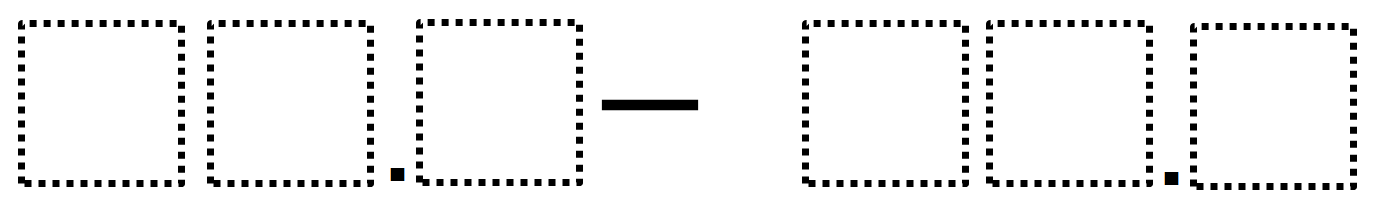Home > Grade 6 > Subtracting Multi-Decimals

# Subtracting Multi-Decimals

Directions: Use the digits 1 to 9, at most one time each, to fill in the boxes so that the difference is as close to 50 as possible. NOTE: The digits used in the difference can be repeated.### Hint

What digit could go in the tens place to be close to 50?

53.1 – 2.9 = 50.2
52.9 – 3.1 = 49.8

Source: Giselle Garica

## Largest Possible GCF #2

Directions: Using the digits 0-9 at most once, fill in the boxes to make the …

1.If the answer is correct then there are too many boxes in the second number for the setup of the problem. What you are suggesting is that it is a 2 digit number with a decimal subtracted by another 2 digit number with a decimal. If you want to fix that the “setup” it should look like this ..

[][].[] – [].[] = ?

Otherwise, the answer key is wrong and should be something like this:
75.1 – 24.9 = 50.2
OR
73.9 – 24.1 = 49.8

2.I told my students they could use 0-9 and then the second number being 3 digits was was ok. Here’s what they came up with:
73.9 – 24.0 = 49.9
74.0 – 23.9 = 50.1

3.My students argued that the directions didn’t specify that digits had to be positive and were able to make exactly 50 with a couple of different combinations:)

35.2-(-14.8)
34.2-(-15.8)
32.4-(-17.6)